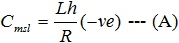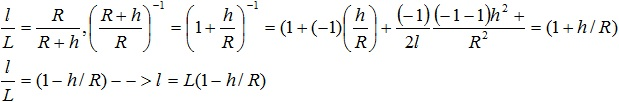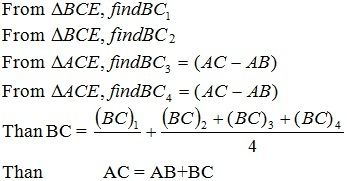Feb 26, 2016

Feb 26, 2016

Feb 26, 2016

# Baseline Measurement and Equipments Used

In triangulation the base line is of prime importance  because it is the only horizontal distance to be measured. It should be measured very accurately since the accuracy of the computed sides of triangulation system depends on it. Length of base line varies form a fraction of (0.5-10) km and a fraction of a mile to 10 miles.

According to the grades of Triangulation

### Baseline Site:

In selecting site for a base line, the following requirement should be considered.

1. The site should be fairly leveled or uniformly sloping or gently undulating.
2. Should be free form obstructions throughout the entire length.
3. Ground should be firmed and smooth.
4. The site should can be such that the whole length can be laid out the extremities of the line being inter-revesible at ground level.
5. The site should be such that well shaped triangle can be obtained in connecting the end stations of the base line to the main triangulation stations.

### Base Net:

A series of triangles connecting a base line to the main triangulation is called a base net. The base should be gradually expended by triangulation as shown figure (i) and (ii)

### Equipment for Baseline Measurements

1. Tape
2. Straining Device
3. Spring balance or weight and pulley
4. Thermometers and a finely divided scale
5. Marking tripod or station rakes
6. Supporting tripods or stakes.

## Field Work & Procedure:

It consists of

1. Setting out party and measuring party.
2. The former places and tripod or stakes in advance of measurement at correct intervals, while actual measurement of the line (base line) is done by the measuring party.

Base line is divided into section of 0.8km to 1.2km [1/2 to ¾ mile in length]
Marking Stakes = 10cm x 10cm (4 in x 4 in)
Supporting Stakes = 2.5 cm x 5cm (1in x 2in)

Another method of measuring the base is to measure the distance b/t the fine marks on two successive tripods.## Reduction to Mean Sea Level:

In geodetic work all the horizontal distances are reduced to their equivalent distance as mean sea level called geodetic distances. If the length of the base is reduced to its equivalent at mean sea level, the computed lengths of all other lines of triangulation sys will corresponds to this level. The mean elev of the base must therefore be determined.

### Purpose:

This correction is required for comparison of various bases.
Given by formulaWhere Cmsl = correction to length L
L = Measured length of base
H = Average height of base above M.S.L
R = mean radius of earth

Proof: Let ‘l’ be the length of the base reduced to M.S.LL = L - Lh/R hence Cmsl = LH/R

The angles measured at the triangulation stations are the horizontal angles and are not effected by the diff of elev of these stations.

## Extension of Baseline:

The length of a base line is usually not greater then (10-20) km i.e, (8-10) miles. As it is not possible to obtain a possible side for a long base, the usually practice is to measure a short base and extend it by means of well conditioned triangles.

### First Method:

Suppose it is required to prolong a base line AB. Let CB, the extremities of the base, is fixed accurately in line AB prolonged by a theologize centered over station A or B such that E and F are dearly visible from it and well shaped triangles are formed.### Second Method:

Another and more common method of extension of a base line is shown in the figure. In this method the base a gradually enlarged through the medium of well proportioned triangles. The base AB is extended to CD be selection suitable stations C and D on the opposite side of AB.